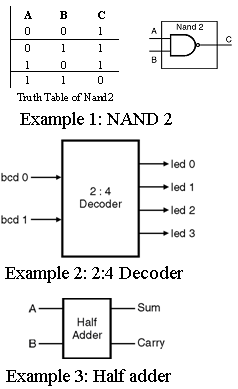Home > VHDL > Introduction > Behavioral modeling

# Behavioral Modeling:

In behavioral modeling sequential execution statements are used. The statements used in this modeling style allowed only inside PROCESSES, FUNCTIONS, or PROCEDURES. Describing a circuit at the behavioral level is very similar to writing a computer program. You have all the standard high-level programming language constructs (like C, BASIC), such as the FOR LOOP, WHILE LOOP, IF THEN ELSE, CASE, and variable assignments. The statements are enclosed in a PROCESS block, and are executed sequentially. When creating a behavioral description of a circuit, you will describe your circuit in terms of its operation over time. Let us take the example of simple NAND2 logic gate as shown in following Fig.4.9.1. In behavioral modeling we must require the behavior of design or simply truth table of design. No need of logical circuit diagram.```  Example 1 : Example of Behavioral style architecture for Entity NAND2.
architecture behave of NAND2 is
begin
process(A, B)
begin
if	(A = '1') and (B = '1') then   Z <= '0';
else 	Z <= '1';
end if;
end process;
end behave;
```

In above example, NAND gate has output z is low if both inputs A and B are high. Otherwise output is high for any other combination of inputs. This behavior of NAND gate is described using if statement. The architecture contains only one statement, called a process statement. It starts at the line beginning with the keyword process and ends with the line that contains end process. All the statements between these two lines are considered part of the process statement

Example 2: 2:4 Decoder

``` architecture behavioral of Decoder is
signal S: bit_vector (3 downto 0);
begin
P1: process (bcd, S)
begin
case bcd is
when "00" => S <= "0001"
when "01" => S <= "0010"
when "10" => S <= "0100"
when "11" => S <= "1000"
end case;
led <= S;
end process P1;
end behavioral;
```
``` Architecture behave of Half-adder is